# Lewis structures

### Lewis structures

#### Lessons

In this lesson, we will learn:
• To construct Lewis (dot and cross) structures using knowledge of electrons shells and the octet rule.
• To use Lewis diagrams to explain the difference between ionic and covalent bonding.
• Some of the exceptions to the octet rule and the significance of this to theories of bonding.

Notes:
• In C11.4.2: Ionic and covalent bonding we saw the two main types of chemical bond: ionic and covalent bonding. These are attractive forces that hold individual atoms together allowing them to form molecules and larger, more complex structures.
We can obviously see that most atoms are just more stable existing in compounds or molecules, such as CH4 or O2, compared to being single isolated atoms, like H or O.
In C11.4.1: Introduction to bonding we mentioned that theories of bonding exist to try to explain why this is true. One theory of bonding is valence bond theory, which uses Lewis structures to show the bonding in simple molecules.

• In Lewis structures, dots and crosses are used to show the valence electrons, where dots or crosses are used for the central atom and the other is used for the atom(s) it is bonding to. They can be used to show covalent and ionic bonding between atoms in molecules and compounds, where electrons are shared or donated/accepted to achieve a full outer shell. Valence means outer shell or highest energy electrons; dont draw core electrons in Lewis structures!
• When drawing these diagrams, look at our lesson Electronic structure: 288 rule for help when finding the number of outer shell electrons. Remember the octet rule, where electrons will try and fill their outer shell with an octet of (eight) electrons.

• Lewis structures for covalent compounds can be drawn step-by-step as below, with CO$_2$ and H$_2$O): as examples:
• Step 1: Write the chemical symbol for the central atom in the molecule and draw a ring around it (this will be the outer electron shell). If the molecule is diatomic, draw either one atom.
• CO2:H2O:• Step 2: Write the symbols of the other atoms equally spaced around it. Draw a ring around each of these so that their rings each have an overlap into the central ring. These rings are the outer electron shells.
• CO2:H2O:• Step 3: For the non-central atoms, fill in their outer shells of electrons using the 288 rule fromElectronic structure: 288 rule (288 rule). Remember to fill up to four electrons individually then start pairing them up. Mark all these electrons using either a dot or a cross. Be consistent with the dots and crosses; if you use crosses for one H atom, use it for all H atoms.

• CO2: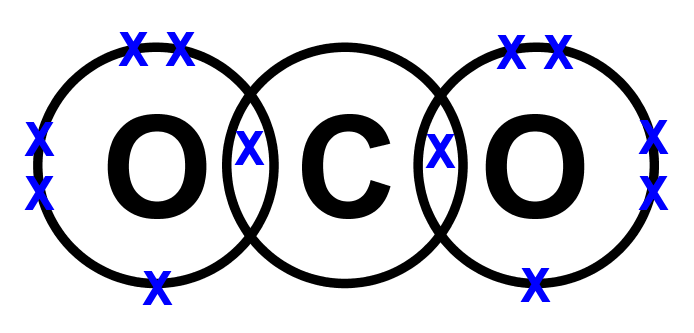H2O: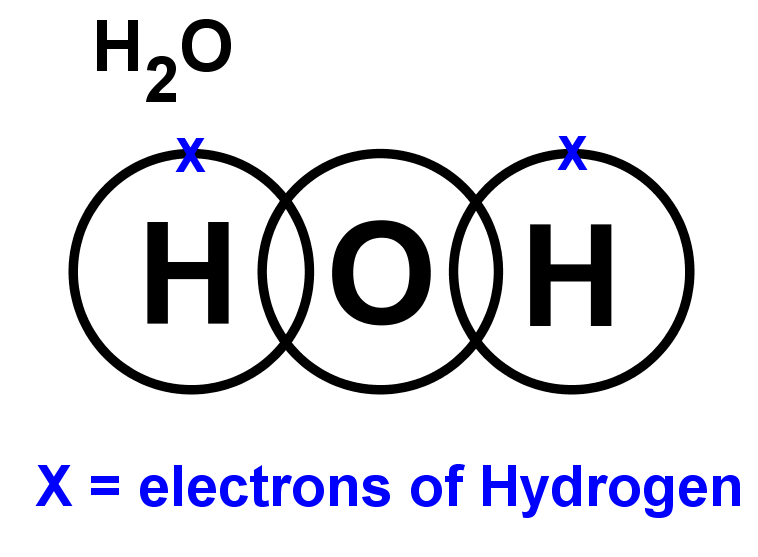• Step 4: LOOK CAREFULLY AT YOUR DIAGRAM. Now make sure any unpaired electrons are placed in the overlap with the central atom.
This is a clue to show how many pairs of electrons (how many covalent bonds) there will be with the central atom. Each of these will be paired with an electron from the central atom too.

• CO2: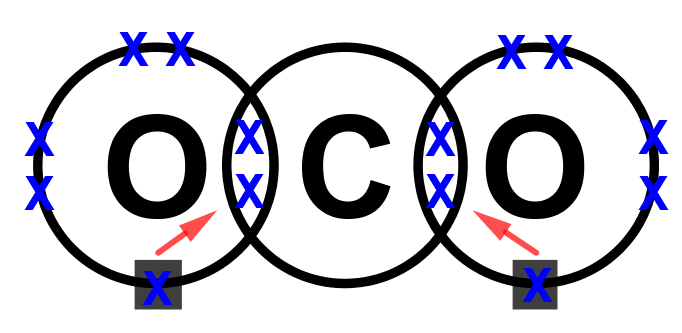H2O: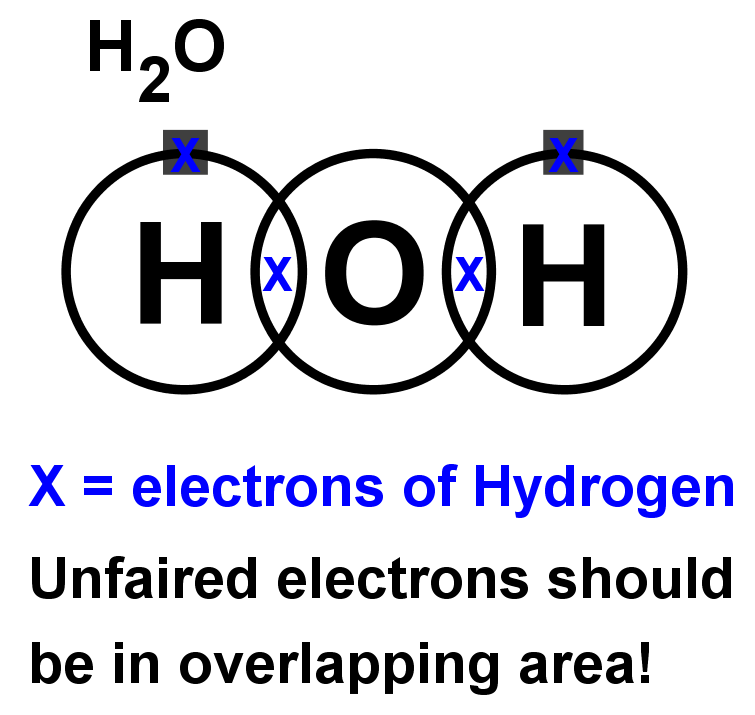• Step 5: Now fill the outer shell of electrons in the central atom. Use the other sign to the one you used with the non-central atom. Start by making pairs of dots and crosses with the electrons of the non-central atoms in the overlapping area. You can only pair dots and crosses in a 1:1 ratio. Now, any extra electrons can be placed outside the overlaps on the outer shell. Remember that if there are more than four electrons in the shell they must now be paired. Electrons in the overlapping area count for both overlapping atoms!

• Step 6: Look carefully at your diagram,. If you have followed these steps, all of your atoms should have exactly 8 electrons (2 if it is hydrogen) in the outer shell. Remember that electrons in the overlaps count for both atoms.

• CO2: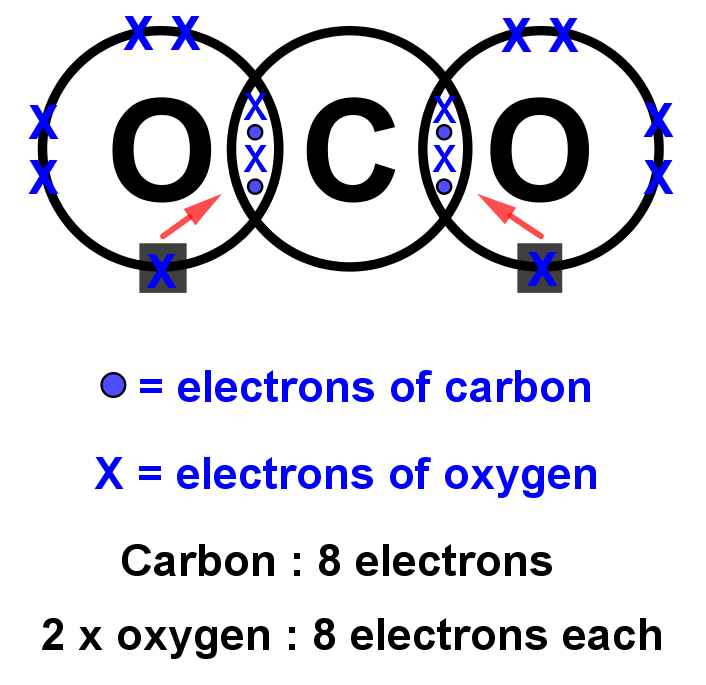H2O: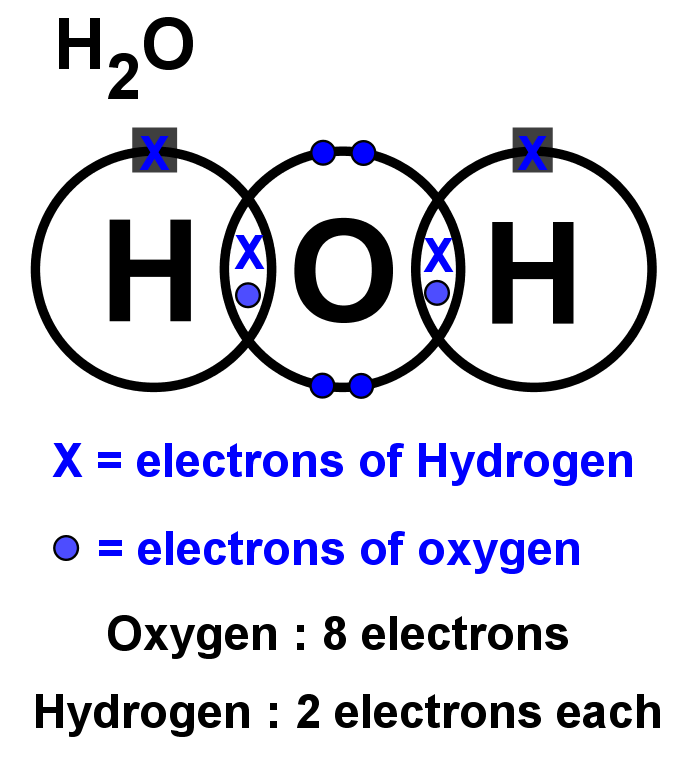• Dot and cross diagrams for ionic compounds can be drawn like this:
• Step 1: Write the chemical symbols of the atoms side by side and draw a ring (the outer shell) around them but keep them separate. They must not overlap like in covalent compounds.

• Step 2: Fill the outer shell with the correct number of electrons for each atom.

• NaCl example: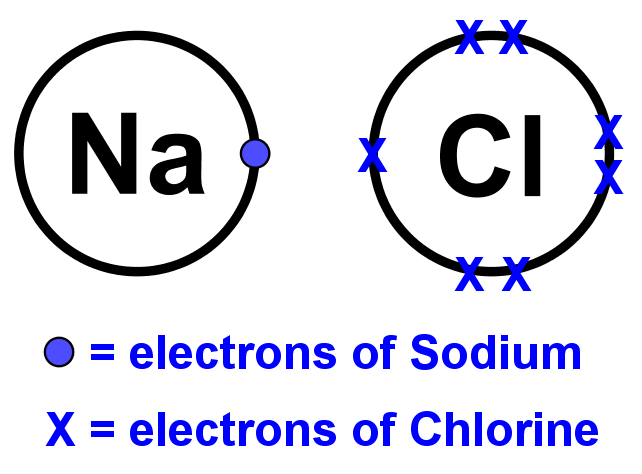• Step 3: Move the electrons from the metal atom(s) to the non-metal atom(s). This should complete the outer shell of the non-metal atom(s) while leaving empty the outer shell of the metal atom(s). The atoms are now ions.

• Step 4: Ions should have square brackets surrounding them with their charge on the top-right corner. This shows an ion has been formed. In order to show an electron was transferred and not shared, keep the original dot/cross signage you used. See below, where the Na outer electron is still a dot on Cl.

• NaCl example: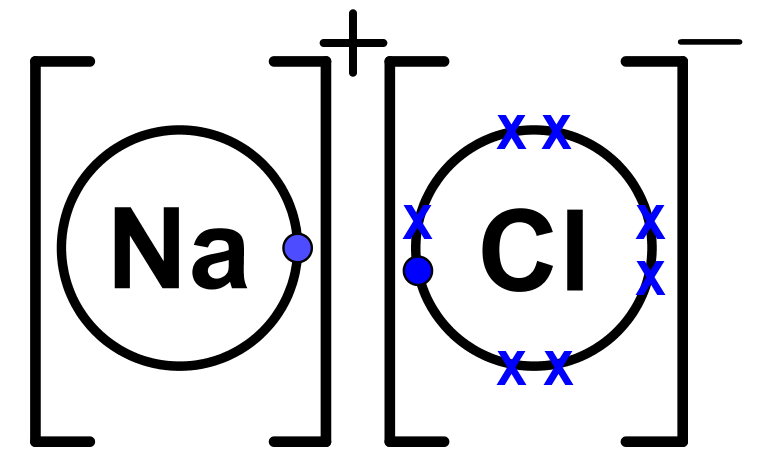• These Lewis diagrams should help to show the difference between bonding in covalent and ionic bonding in terms of electrons:
• Covalent bonding involves a sharing of electrons to complete outer shells in both atoms.
• Ionic bonding involves a discrete transfer of electrons usually from a metal atom to a non-metal atom, which also leads to complete outer shells.

• Building Lewis structures using the octet rule is applying a theory of chemical bonding. These theories are made to try and explain what we observe and to make predictions that can be tested, but there are exceptions to the octet rule.

• For example, the molecules NO (nitric oxide) and BF3 (boron trifluoride):
• Nitric oxide (NO) would have a Lewis structure beginning with six electrons around oxygen, and five electrons around nitrogen. This means nitrogen needs three more electrons and oxygen two to satisfy the octet rule. This cannot be satisfied by:
• A single bond (one pair of electrons) between the two atoms; it leaves only six electrons on nitrogen and seven on oxygen.
• A double bond (or two pairs of electrons) between the two atoms; it leaves only seven electrons on nitrogen and eight on oxygen.
• A triple bond between the two atoms; oxygen now has nine electrons in the outer shell, violating the octet rule.

• Boron trifluoride (BF3) would have a Lewis structure beginning with three electrons around boron and seven around all three fluorine atoms each. The octet rule cannot be satisfied for boron. It has only three electrons, and with one covalent bond each to the three fluorine atoms, it is still has only six electrons when it should have eight.

If the theory behind Lewis structures was complete and totally accurate, neither of these two molecules should exist. The fact that they do shows the theory is not totally accurate!
• Introduction
Introduction to dot and cross diagrams
a)
Lewis structures: introduction.

b)
How to draw covalent Lewis structures.

c)
How to draw ionic Lewis structures.

d)
Exceptions to the octet rule.

• 1.
Draw Lewis structures for simple covalent compounds.
Draw dot and cross structures for the following molecules:
a)
HCl

b)
N$_2$

c)
NH$_3$

d)
AlCl$_3$

e)
NH$_{4}$+

• 2.
Draw Lewis structures for simple ionic compounds.
Draw dot and cross structures for the following molecules:
a)
KCl

b)
Na$_2$O

c)
CaCl$_2$